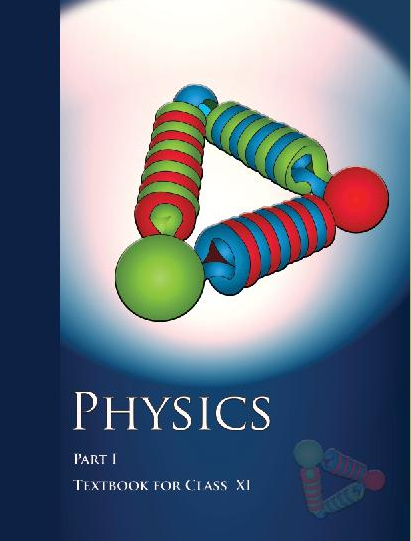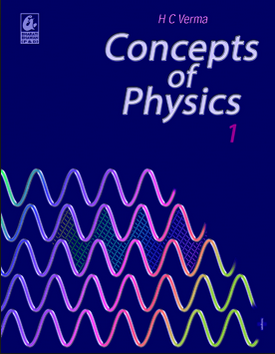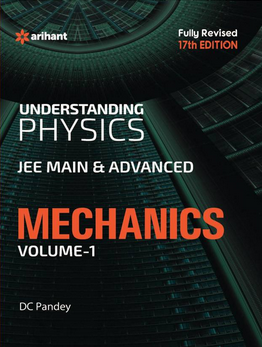# Laws of motion   Share

## What is Laws of motion

Laws of motion are the laws given by Newton regarding the motion of objects. You are already familiar with the laws of motion as you apply or observe these laws on a daily basis in various daily activities. This chapter helps you to understand these laws in mathematical forms with some suitable examples. For example, you know that if you apply the same force on an elephant and on football as shown in the below figure then the football will move more quickly than the elephant.This is nothing but Newton's 2nd law which mathematically says

F=ma where F= Force on the body, m=mass of the body and a= acceleration of the body.

So as a mass of football is less than that of an elephant So for same force football will have more acceleration, and will move quickly.

Laws of motion is a very basic and important chapter from the mechanics part of physics. It is very important to master the concepts from this chapter at an early stage as this forms the basis of your preparation for all competitive exam. This chapter is very important because it will test your knowledge of the chapter Kinematics, and it will be also useful and crucial for you for solving questions from the next chapters namely Work Energy and Power, Rotational Motion and to some extent gravitation. This chapter will also tell you how important these laws are in our daily life as well as studying physics.

Prepare Online for JEE Main/NEET

Crack JEE 2021 with JEE/NEET Online Preparation Program

## Notes on Laws of motion-

So we will discuss step by step about important topics from this chapter followed by an overview of this chapter.

Then we will understand important formulas from this chapter. Remembering these formulas will increase your speed while question-solving.

## Laws of motion Topics

• Force and Inertia
• Newton's Law of motion- Newton's First Law, Newton's Second Law, Newton's Third Law
• Momentum, Impulse
• Law of conservation of linear momentum and applications of linear momentum
• Equilibrium of concurrent forces
• Kinetic and Static friction, laws of friction, rolling friction
• Dynamics of uniform circular motion, Centripetal force, and applications of Centripetal force.

## Laws of motion Overview

In this chapter, we will learn about the various force and laws of motion. As you know that if you have to move stationary body you will have to apply force, similarly if you have to stop a moving body then also you have to apply force. This is nothing but Newton's 1st law which you will learn in this chapter and which says every object will remain at rest or in uniform motion until we don't apply any external force to change its state. And very important and interesting law which is third laws of motion which states that for every action (force) in nature there is an equal and opposite reaction. We see various examples and application of Newton's laws of motion in our ur day to day activities. Walking, Bouncing Of Ball, Rocket Propulsion all have an application of Newton's third law. In this chapter, you will also learn about Inertia and its type, Momentum, Force and its types with example and Impulse, friction and its types, etc. In this chapter, you will deal with forces like  Weight, Reaction or Normal Force, Tension, Spring force, etc. You will also be able to add these forces with the help of various force vector addition laws like parallelogram law of force and polygon law of forces to get resultant forces. You will learn about friction which is nothing but an Opposing Force which is parallel to the surface and opposite to the direction of Relative Motion.

## Laws of motion Formulas

• For equilibrium,

• Lami’s theorem-• Spring force-

• Newton's 2nd Law

F=ma

• Newton's 3rd Law

• Linear Momentum

• Impulse=

• Impulse-Momentum Theorem-

• Centripetal Force=

• Kinetic friction force =

• Limiting Friction force=

## How to prepare Laws of motion

First, you should have good command over the concepts of the Laws of motion and you should also know how to apply them well at the time of the entrance exam or while solving questions. Please try to understand each concept from this chapter, with the help of theory, questions with solutions and video lectures on each important concept. For each concept practice enough problems so that you have a thorough understanding of the concept. Solve all the questions at home with proper concentration and try to do all calculation by yourself without seeing the solution first.

How to Solve Laws of motion questions.

1. Choose a convenient system.

2.  Draw a diagram which schematically shows the various parts of a system.

3. Draw the free-body diagram of each part of the system.

4. In a free-body diagram, you must include information about forces (their magnitudes and directions).

5. The rest should be treated as unknowns or to be determined using laws of motion.

6. Apply  Newton's third law wherever necessary.

If a system is in equilibrium or all the forces working on a body are Concurrent Forces then calculation in the questions became easy. Problems related to Recoiling of Gun, Firing of Bullet, Rocket Propulsion needs a clear understanding of the concept and neat and clean calculation to avoid negative marking. Question on the friction gets more and more complicated when we involve more blocks in contact, inclined planes, pulleys, etc, variable external force, etc. But you can solve these questions with ease with proper study.

## Laws of motion Tips

1. This chapter is more of a concept based chapter there is very little to memorize, hence practice is the key here for getting a good hold on this chapter.

2. Lots of question will ask you to draw FBD so draw clear FBD and then solve the questions. This will increase your accuracy.

3. Make a plan to prepare for this chapter and Stick to a Timetable.

4. Study the concept first and then start solving Laws of motion questions. Don't go through question directly without knowing the concept.

5. Solve previous year question of various exam from this chapter.

6. Use smart methods to solve questions.

Because as said by Matshona Dhliwayo

“Work hard, and you will earn good rewards.

Work smart, and you will earn great rewards.

Work hard and work smart, and you will earn extraordinary rewards"

## Laws of motion Books

For Laws of motions, chapter concepts in NCERT are enough but you will have to practice lots of questions including previous year questions and you can follow other standard books available for competitive exam preparation like Concepts of Physics (H. C. Verma) and Understanding Physics by D. C. Pandey (Arihant Publications).

## Physics Chapter wise Notes For Engineering and Medical Exams

 Chapters No. Chapters Name Chapter 1 Physics and Measurement Chapter 2 Kinematics Chapter 4 Work Energy and Power Chapter 5 Rotational Motion Chapter 6 Gravitation Chapter 7 Properties of Solids and Liquids Chapter 8 Kinetic theory of Gases Chapter 9 Thermodynamics Chapter 10 Oscillations and Waves Chapter 11 Electrostatics Chapter 12 Current Electricity Chapter 13 Magnetic Effects of Current and Magnetism Chapter 14 Electromagnetic Induction and Alternating currents Chapter 15 Electromagnetic Waves Chapter 16 Optics Chapter 17 Dual Nature of Matter and Radiation Chapter  18 Atoms And Nuclei Chapter 19 Electronic devices Chapter 20 Communication Systems Chapter 21 Experimental skills

### Topics from Laws of motion

• Force and Inertia,Newton's First Law of motion ( JEE Main ) (15 concepts)
• Momentum,Impulse ( JEE Main ) (4 concepts)
• Newton's Second Law of motion;Newton's Third Law of motion. ( JEE Main ) (2 concepts)
• Law of conservation of linear momentum and its applications ( JEE Main ) (4 concepts)
• Equilibrium of concurrent forces. ( JEE Main ) (26 concepts)
• Static and Kinetic friction, laws of friction, rolling friction. ( JEE Main ) (32 concepts)
• Dynamics of uniform circular motion: Centripetal force and its applications. ( JEE Main ) (27 concepts)
• Friction ( JEE Main ) (20 concepts)
• Newtons First Law ( JEE Main ) (5 concepts)
• Newtons 2nd and 3rd Laws of motion ( JEE Main ) (2 concepts)
• Applications of Newtons 2nd and 3rd Laws of motion ( JEE Main ) (12 concepts)
• Dynamics of uniform circular motion ( JEE Main ) (8 concepts)

### Important Books for Laws of motion

•••Exams
Articles
Questions